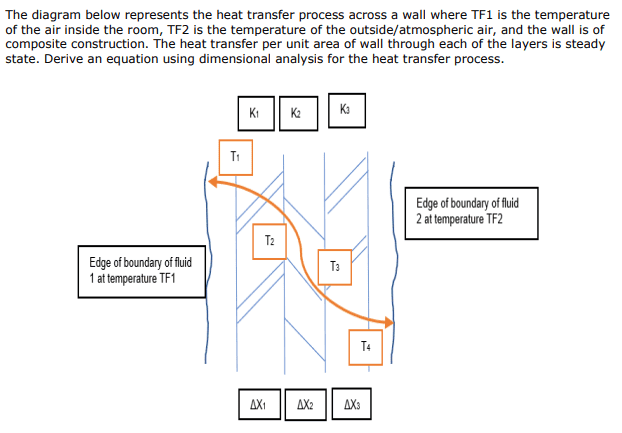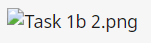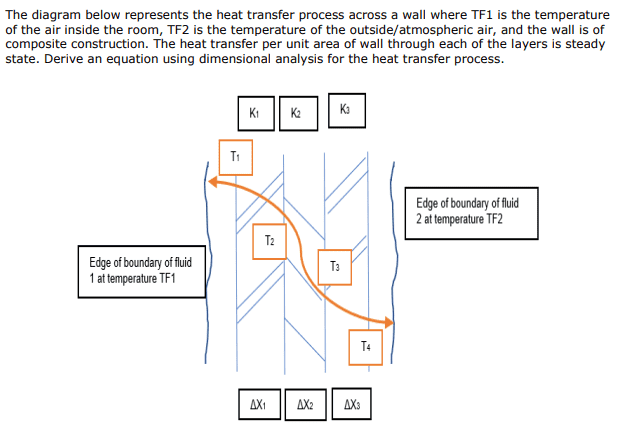# Dimensional analysis homework

Ben_Walker1978
Homework Statement:
Dimensional Analysis
Relevant Equations:
q = U A dT
Hello,

New to the forum. I am looking for some help with some work.

It is dimensional Analysis, i have done Dimensional analysis with the analysis bridge before. But i am stuck with the question below and trying to learn it. Any help much appreciated.

I have the equation q = U A dT. But i am now stuck.

Attached question below.Last edited:

Homework Helper
I only see, no picture ...

•Ben_Walker1978
Ben_Walker1978
I only see View attachment 257037, no picture ...Ben_Walker1978

Homework Helper
What is the idea ? Solving the lot ? If so, you need to work towards N equations with N unknowns. What are the knowns, what are the unknowns ? Make a list...

•Ben_Walker1978
Ben_Walker1978
What is the idea ? Solving the lot ? If so, you need to work towards N equations with N unknowns. What are the knowns, what are the unknowns ? Make a list...

Yes solve the lot.

The knows are TF1, TF2, K1, K2, K3??

Homework Helper
I should have written: make three lists: one of knowns, one of unknowns and one of equationsDon't see no equations with k in them ?

What about U ? How many of them ?

What about these edges ? Is TF1 = T1 or is there an equation involving them ?
Same for TF2 and T4

•Ben_Walker1978
Ben_Walker1978
TF1-TF2 = dt in the equation q = U A dT . U = k/s. So is that T?

Homework Helper
In the problem statement there is no mention of any heat conduction resistance of the boundary layer, so my impression is that the best you can do is to assume that TF1 = T1

Ben_Walker1978
Ok. So how do i then derive an equation using dimensional analysis? One i know i can copy the answer and method from this and then practice.

Homework Helper
Perhaps by writing down the dimensions of the variables involved ? In particular the ones in boxes, and also your Q

•Ben_Walker1978
Homework Helper
The knowns are TF1, TF2, K1, K2, K3??
I would take the ##\Delta x## as known, too.

•Ben_Walker1978
Homework Helper
Gold Member
2022 Award
I have the equation q = U A dT
Isn't that the equation you are trying to obtain through dimensional analysis (but in terms of the given variables ΔT, Δx etc.)?
If so, start by stating the dimensionality of each of the input variables and of the dependent variable (heat flow rate).

One thing is unclear in the question: what to do about area. You are not given a variable for the area, so you could define that as another input variable, A, or take the dependent variable as being flow per unit area.

•Ben_Walker1978
Mentor
You also need to include the inside and outside convective heat transfer coefficients in your known list.

•Ben_Walker1978
Homework Helper
Gold Member
2022 Award
You also need to include the inside and outside convective heat transfer coefficients in your known list.
The question seems strange. How is dimensional analysis relevant to constructing the complete equation for transfer through three walls? Surely it only assists in finding the equation (up to an unknown multiplicative constant) through one wall.
Maybe this is only the first part of a multipart question.

•Ben_Walker1978
Ben_Walker1978
So the Known's are TF1, TF2, K1, K2, K3 and \Delta x. q = U A dT is an equation i found from research online, I'm not sure if this is the equation i need to achieve. I am not sure how to state the dimension ally of each of the input variables and of the dependent variable. I am new trying to learn this, so appreciate all the help i am receiving. I want to be able to complete the above so i can write it down, learn and remember it for the future.

Homework Helper
Gold Member
2022 Award
So the Known's are TF1, TF2, K1, K2, K3 and \Delta x. q = U A dT is an equation i found from research online, I'm not sure if this is the equation i need to achieve. I am not sure how to state the dimension ally of each of the input variables and of the dependent variable. I am new trying to learn this, so appreciate all the help i am receiving. I want to be able to complete the above so i can write it down, learn and remember it for the future.
Yes, I would say you are to derive q = U A dT or something equivalent.
For this question you need to represent the dimensions length L, temperature Θ, time T and heat. I don't think there is a standard single symbol for heat since it is , after all, a form of energy, but you could borrow Q for the occasion.
You need to figure out the dimensionality of k, perhaps from whatever units it is expressed in.

Ben_Walker1978
Ok. I thought it was deriving to something like q = U A dT. I have been looking online to try to find how to complete this. But have had no luck. Could anyone complete this for me so i can see how its done, then understand how to do it. Would be very grateful.

Homework Helper
Gold Member
2022 Award
Ok. I thought it was deriving to something like q = U A dT. I have been looking online to try to find how to complete this. But have had no luck. Could anyone complete this for me so i can see how its done, then understand how to do it. Would be very grateful.
Do you know how to write physical variables in terms of dimensional constituents? E.g. force is MLT-2?

•Ben_Walker1978
Homework Helper
Could anyone complete this for me

Not how PF works. You have the problem, we help.
You have a flow of energy from hot to cold that has to evercome three resistances. Almost like an electric series circuit of three resistances.

I still didn't see the lists I mentioned ...

•Pi-is-3 and Ben_Walker1978
Ben_Walker1978
Knowns = Tf1, Tf2, K1, K2, K3, $$\Delta x$$
Equation = q = U A dT
Unknowns = T1,T2,T3,T4
Is this the correct lists?

Mentor
Knowns = Tf1, Tf2, K1, K2, K3, $$\Delta x$$
Equation = q = U A dT
Unknowns = T1,T2,T3,T4
Is this the correct lists?
Like I said, you also need ##h_{left}## and ##h_{right}## as knowns.

I still don't see how dimensional analysis would be used to solve this.

•Ben_Walker1978
Homework Helper
Knowns = Tf1, Tf2, K1, K2, K3, ##\Delta x##
Equation = q = U A dT
Unknowns = T1,T2,T3,T4
Is this the correct lists?
Knowns: not ##\Delta x## but ##\Delta x_1##, ##\Delta x_2##, ##\Delta x_3##
Unknowns: Q as well . (*)
Equations:

You need as many of those as there are unknowns.
And if a new variable appears, you will also need a new equation, e.g.
Ui ?​
dTi ?​

I suggested to use TF1 = T1 and TF2 = T4

(@Chestermiller : the exercise shows a boundary but does not give any info whatsoever. OP indicates this is a first encounter with the subject, so I propose to keep it simple)

You have been given k1 etc. so you need to find out what the relation is between ki and Ui

(*) Is it clear to you that 'Steady state' means Q1 = Q2 = Q2 ?

The problem statement suggests you are expected to calculate the heat flow per unit of area, i.e. Q/A , which has dimension energy/time/length2.​

•Ben_Walker1978
Homework Helper
I found a nice example when I googled 'heat flow through wall'

•Ben_Walker1978
•